Next: The extended Boolean model Up: Boolean retrieval Previous: A first take at   Contents   Index

# Processing Boolean queries

How do we process a query using an inverted index and the basic Boolean retrieval model? Consider processing the simple conjunctive query :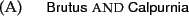over the inverted index partially shown in Figure 1.3 (page). We:

1. Locate Brutus in the Dictionary
2. Retrieve its postings
3. Locate Calpurnia in the Dictionary
4. Retrieve its postings
5. Intersect the two postings lists, as shown in Figure 1.5 .
The intersection is the crucial one: we need to efficiently intersect postings lists so as to be able to quickly find documents that contain both terms. (This operation is sometimes referred to as merging postings lists: this slightly counterintuitive name reflects using the term merge algorithm for a general family of algorithms that combine multiple sorted lists by interleaved advancing of pointers through each; here we are merging the lists with a logical AND operation.)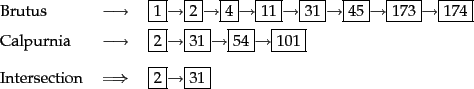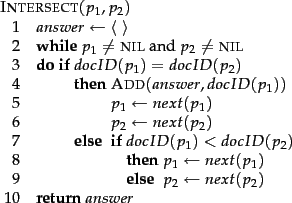There is a simple and effective method of intersecting postings lists using the merge algorithm (see Figure 1.6 ): we maintain pointers into both lists and walk through the two postings lists simultaneously, in time linear in the total number of postings entries. At each step, we compare the docID pointed to by both pointers. If they are the same, we put that docID in the results list, and advance both pointers. Otherwise we advance the pointer pointing to the smaller docID. If the lengths of the postings lists are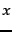and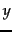, the intersection takes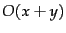operations. Formally, the complexity of querying is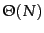,where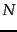is the number of documents in the collection.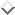Our indexing methods gain us just a constant, not a difference in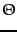time complexity compared to a linear scan, but in practice the constant is huge. To use this algorithm, it is crucial that postings be sorted by a single global ordering. Using a numeric sort by docID is one simple way to achieve this.

We can extend the intersection operation to process more complicated queries like: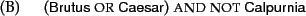Query optimization is the process of selecting how to organize the work of answering a query so that the least total amount of work needs to be done by the system. A major element of this for Boolean queries is the order in which postings lists are accessed. What is the best order for query processing? Consider a query that is an AND of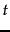terms, for instance: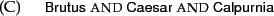For each of theterms, we need to get its postings, then AND them together. The standard heuristic is to process terms in order of increasing document frequency: if we start by intersecting the two smallest postings lists, then all intermediate results must be no bigger than the smallest postings list, and we are therefore likely to do the least amount of total work. So, for the postings lists in Figure 1.3 (page), we execute the above query as: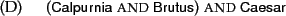This is a first justification for keeping the frequency of terms in the dictionary: it allows us to make this ordering decision based on in-memory data before accessing any postings list.

Consider now the optimization of more general queries, such as: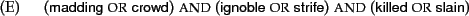As before, we will get the frequencies for all terms, and we can then (conservatively) estimate the size of each OR by the sum of the frequencies of its disjuncts. We can then process the query in increasing order of the size of each disjunctive term.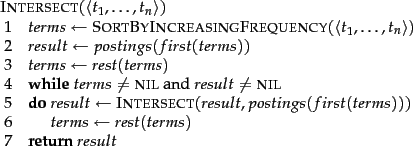For arbitrary Boolean queries, we have to evaluate and temporarily store the answers for intermediate expressions in a complex expression. However, in many circumstances, either because of the nature of the query language, or just because this is the most common type of query that users submit, a query is purely conjunctive. In this case, rather than viewing merging postings lists as a function with two inputs and a distinct output, it is more efficient to intersect each retrieved postings list with the current intermediate result in memory, where we initialize the intermediate result by loading the postings list of the least frequent term. This algorithm is shown in Figure 1.7 . The intersection operation is then asymmetric: the intermediate results list is in memory while the list it is being intersected with is being read from disk. Moreover the intermediate results list is always at least as short as the other list, and in many cases it is orders of magnitude shorter. The postings intersection can still be done by the algorithm in Figure 1.6 , but when the difference between the list lengths is very large, opportunities to use alternative techniques open up. The intersection can be calculated in place by destructively modifying or marking invalid items in the intermediate results list. Or the intersection can be done as a sequence of binary searches in the long postings lists for each posting in the intermediate results list. Another possibility is to store the long postings list as a hashtable, so that membership of an intermediate result item can be calculated in constant rather than linear or log time. However, such alternative techniques are difficult to combine with postings list compression of the sort discussed in Chapter 5 . Moreover, standard postings list intersection operations remain necessary when both terms of a query are very common.

Exercises.

• For the queries below, can we still run through the intersection in time, whereandare the lengths of the postings lists for Brutus and Caesar? If not, what can we achieve?
1. Brutus and not Caesar
2. Brutus or not Caesar

• Extend the postings merge algorithm to arbitrary Boolean query formulas. What is its time complexity? For instance, consider:
c.
(Brutus OR Caesar) AND NOT (Antony OR Cleopatra)
Can we always merge in linear time? Linear in what? Can we do better than this?

• We can use distributive laws for and and or to rewrite queries.
1. Show how to rewrite the query in Exercise 1.3 into disjunctive normal form using the distributive laws.
2. Would the resulting query be more or less efficiently evaluated than the original form of this query?
3. Is this result true in general or does it depend on the words and the contents of the document collection?

• Recommend a query processing order for
d.
(tangerine OR trees) AND (marmalade OR skies) AND (kaleidoscope OR eyes)
given the following postings list sizes:
Term Postings size
eyes 213312
kaleidoscope 87009
skies 271658
tangerine 46653
trees 316812

• If the query is:
e.
friends AND romans AND (NOT countrymen)
how could we use the frequency of countrymen in evaluating the best query evaluation order? In particular, propose a way of handling negation in determining the order of query processing.

• For a conjunctive query, is processing postings lists in order of size guaranteed to be optimal? Explain why it is, or give an example where it isn't.

• Write out a postings merge algorithm, in the style of Figure 1.6 (page), for anORquery.

• How should the Boolean queryAND NOTbe handled? Why is naive evaluation of this query normally very expensive? Write out a postings merge algorithm that evaluates this query efficiently.Next: The extended Boolean model Up: Boolean retrieval Previous: A first take at   Contents   Index